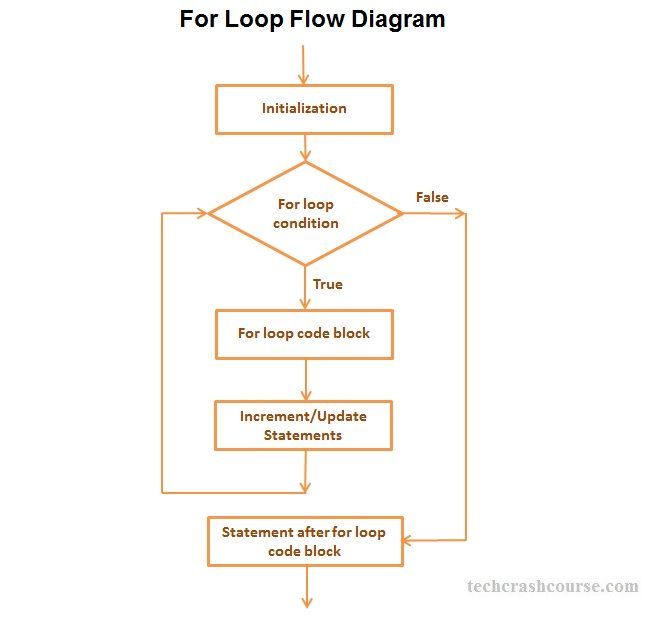# C++ For Loop

The for loop in C++ is used to execute execute some statements repetitively until the given condition is true. The for loop is mainly used to perform repetitive tasks.

Syntax of for Loop
```for(initialization; condition; update) {
/* code to be executed */
}
```
For Example:
```for(i = 1; i <= 10; i++){
cout << i;
}
```
Above for loop prints integers from 1 to 10;

## For Loop Syntax Description

• Initialization : The Initialization statement allows us to declare and initialize any loop variables. It is executed only once at the beginning of the for loop.

• Condition : After initialization, condition expression is evaluated. It is a boolean expression which decides whether to execute loop's body or terminate for loop. if condition expression evaluates to true, the for loop code block gets executed otherwise it will terminate for loop and control goes to the next statement after the for loop.

• Update : After code block of for loop gets executed, control comes back to update statements. It allow us to modify any loop variable for next iteration of loop. This step is mainly used for updating loop control variable.

• All three fields of a for loop are optional. They can be left empty, but in all cases the semicolon signs between them are required.

## Control Flow Diagram of for loopHow for loop works
• The Initialization statement will be executed first. We can declare and initialize any number of loop control variables here.
• After the Initialization statement the condition expression is evaluated. If the value of condition expression is true then code block of for loop will be executed otherwise the loop will be terminated.
• After execution of the code block of for loop control goes to update statements of the loop statement which modifies the loop control variables.
• After modifying control variables in update statements, control again goes to condition expression.
• For loop iteration continues unless condition expression becomes false or for loop gets terminated using break statement.

## C++ for Loop Example Program

```#include <iostream>
using namespace std;

int main(){
int N, i, sum=0;

cout << "Enter a positive number\n";
cin >> N;
// Using for loop to find the sum
// of all integers from 1 to N
for(i=1; i <= N; i++){
sum+= i;
}

printf("Sum of all numbers from 1 to %d = %d", N, sum);

return 0;
}
```

Output
```Enter a positive number
6
Sum of all numbers from 1 to 6 = 21
```
In above program, we first take a number N as input from user. Now, we have to find sum of all numbers from 1 to N. We are using a for loop to iterate from 1 to N and add each number to variable sum.
For Loop Facts
• The condition_expression in for loop is a boolean expression. It must evaluate to true or false value(In C++, all non-zero values are considered as true and zero is considered as false).

• Initialization statement, Condition expression and Update statement are all optional in for loop.

• You can use multiple initialization statements and update statements inside for loop.
For Example:
for(i=0, j=50; i < 100; i++, j--)

• Opening and Closing braces are not required for single statement inside for loop code block.
For Example:
for(i = 0; i < 100; i++)
sum+= i;

• We can also use infinite for loops like for(;;), which will never terminate. You should add terminating condition using break statement in order to terminate infinite for loop.
For Example:
for(;;){
if(.....){
break;
}
}